# A New Approach to ICSE Physics Part 1 Class 9 Solutions Law of Motion

## A New Approach to ICSE Physics Part 1 Class 9 Solutions Law of Motion.

These Solutions are part of A New Approach to ICSE Physics Part 1 Class 9 Solutions. Here we have given A New Approach to ICSE Physics Part 1 Class 9 Solutions Law of Motion.

UNIT I

Practice Problems 1
Question 1.
Calculate the velocity of a body of mass 0.5 kg, when it has a linear momentum of 5 Ns.
Mass of body = m = 0.5 kg
Linear momentum = P = 5 Ns
Velocity of body = v = ?
We know P = mv
$$y=\frac{P}{m}=\frac{5}{0.5}$$
v = 10 ms-1

Question 2.
An electron of mass 9 x 10-31 kg is moving with a linear velocity of 6×107 ms-1. Calculate the linear momentum of electron.
Mass of electron = m = 9 ×10-31 kg
Velocity of electron = v = 6 × 107 ms-1
Momentum of electron = P = ?
We know, P = mv
P = 9.1 x 10-31 x 6 x 107
P = 54 x 10-24 kg ms-1 or Ns
= 54 x 10-24 kg ms-1 Ns

Question 3.
A body of mass 200 g is moving with a velocity of 5 ms-1. If the velocity of the body changes to 17 ms-1, calculate the change in linear momentum of the body.
Mass of body = m = 200 g = 0.2 kg
Velocity = v1 = 5 ms-1; Velocity = v2 = 17 ms-1
Change in linear momentum of body
= mv2 – mv1= m (v2 – v1)
= 0.2(17-5)
= 2.4 Ns or kg ms-1

Question 4.
A motorcycle of mass 100 kg is running at 10 ms-1. If its engine develops an extra linear momentum of 2000 Ns, calculate the new velocity of motorcycle.
Mass of motor cycle = m = 100 kg
Velocity of motor cycle = v1= 10 ms-1
Momentum of motor cycle = mv1 = 100 x 10 = 1000 Ns
When engine develops an extra linear momentum of2000 Ns
Then total momentum of motor cycle = 1000 + 2000 = 3000 Ns
Let v2 = new velocity of the motor cycle.
Total momentum of motor cycle = mv2
3000 = 100 x v2
$$v_{2}=\frac{3000}{100}=30 \mathrm{ms}^{-1}$$

Practice Problems 1:
Question 1.
A car initially at rest, picks up a velocity of 72 kmh-1 in 20 seconds. If the mass of the car is 1000 kg, find (1) Force developed by its engine
(2) Distance covered by the car.
Initial velocity = u = 0
Final velocity = v = 72 kmh-1
$$v=72 \times \frac{5}{18} \mathrm{ms}^{-1}=20 \mathrm{ms}^{-1}$$
Mass of car = m = 1000 kg
Time = t = 20 s

(1) v = u + at
20 = 0 + a (20)
(Accelaration ) a=$$\frac{20}{20}$$=1 ms-2
Now, F = ma
F = 1000 x 1 = 1000 N

(2) Distance covered by car = S = ?
S=ut + $$\frac{1}{2}$$ at2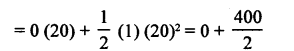S = 200 m

Question 2.
A golfer hits a ball at rest, such that the contact between the ball and golf stick is for 0.1 s. If the golf ball covers a linear distance of 400 m in 2 s, find the magnitude of force applied. Mass of golf ball is 50 g.
Distance covered by ball = 400 m
Time taken = 2 s
Distance 400
∴ Uniform Velocity of the ball = $$\frac{\text { Distance }}{\text { Time }}=\frac{400}{2}$$ =200ms-1
Now initial velocity of the ball = u = 0
Time for which force acts on the ball = t = 0.1 s
Final velocity of the ball after the force stops acting on it
= v = 200 ms-1
Using, v = ut + at
We have, 200 = 0 + a (0.1)
a = $$\frac{200}{0.1}$$ = 2000ms-2
Mass of the ball = m = 50 g = 0.05 kg
Force acting on the ball = F = ma
F = 0.05 x 2000
F = 100N

Practice Problems 2:
Question 1.
A car of mass 800 kg, moving at 54 kmh-1 is brought to rest over a distance of 15 m. Find the retarding force developed by the brakes of the car.
Mass of car = m = 800 kg
Initial velocity of car = u – 54 kmh-1Final velocity of car = v = 0
Distance covered = S = 15 m
Retarding force = F = ?
v2 – w2 = 2a S
(0)2-(15)2 = 2a(15)
30 a = -225
a= $$-\frac{225}{30}$$
a = -7.5 ms-2
F = ma = (800) (-7.5)
F = -6000N

Question 2.
A cricket player holds a cricket ball of mass 100 g by moving his hands backward by 0.75 m. If the initial velocity of the ball is 108 kmh-1, find the retarding force applied by the player.
Mass of ball – m = 100 g = 0.1 kg
Distance covered = S = 0.75 m
Initial velocity of ball = u = 108 kmh-1Final velocity of car = v = 0
Retarding force = F = ?
v2 – w2 = 2a S
(0)2-(30)2 = 2a (0.75)
1.5 a = -900Retarding force = F = ma
F = 0.1 x (-600)
F = -60 N

Practice Problems 3:

Question 1.
A force of 600 dynes acts on a glass ball of mass 200 g for 12 s. If initially the ball is at rest,
find
(1) Final velocity
(2) Distance covered.
F = 600 dyne = 600 g cms-2
m = 200 ga 3 cms-2
Also time = t= 12 s
Initial velocity = u = 0
(1)
Final velocity = v = ?
v = u + at
v = 0 + 3 (12)
v = 36 cms-1
(2)
Distance covered = S = ?
v2 – w2 = 2a s
(36)2-(0)2 = 2(3)s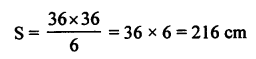Question 2.
A bullet of mass 30 g, and moving with a velocity x hits a wooden target with a force of 187.5 N. If the bullet penetrates 80 cm, find the value of x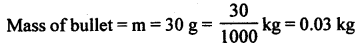Force = F = 187.5 N
Acceleration = a — ?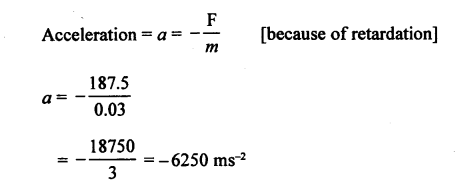Initial velocity = u = x
Final velocity = v = ?S=0.8m
v2 – u2=2asQuestion 3.
A car of mass 1000 kg develops a force of 500 N over a distance of 49 m. If initially the car is at rest find (1) Final velocity (2) time for which it accelerates.
Mass of car = m = 1000 kg
Force = F = 500 NInitially velocity = u=0
Distance covered = S = 49 m
Final velocity = v = ?
Time = t = ?
(1) v2 – m2 = 2a S
v2 – (0)2 = 2 (0.5) (49)
v2 = 49
v = 7 ms-1

(2) v = u + at
7 = 0 + 0.5t
0.5 t=7
t=$$\frac{7}{0.5}$$=14s

EXERCISE 1

Question 1.
(a) Define force.
(b) State four effects which a force can bring about. Give two examples in each case.
(a) Force : It is defined as an external cause which changes or tends to change the state of rest or uniform motion of a body in a straight line.
(b) Effects of force : A force can bring about the following effects.

1. Force can set a stationary body into motion.
For example :

1. A player can set a ball (at rest) in motion by hitting it with suitable material like hockey.
2. A magnet can move an iron nail.

2.  Force can stop the moving bodies.
For example :

1. A speeding car is stopped by the force of friction of brakes.
2. A rolling football stops because of the force of friction from the ground.

3. Force can change the speed or direction of a moving body.
For example :

1. A stone projected vertically upwards changes its speed and direction of motion because of the force of gravity.
2. A moving bicycle starts running faster, when more force is applied on its peddles.

4. Force can change the dimensions of a body.
For example :

1. Lenght of a rubber band increases, when stretching force is applied on it.
2. We clay can be moulded in any shape by applying a force with hands.

Question 2.
What do you understand by the following terms?
(a) Contact forces (b) Non-contact forces
(a) The forces which act on bodies when they are in actual contact, are called the contact forces. Two examples are as below:

1. Force of friction
2. Force of tension.

(b) The forces which act on bodies without being physically touched, are called the non-contact forces or the forces at a distance. The gravitational force, electrostatic force and magnetic force are the non-contact forces.

Question 3.
(a) What do you understand by the term inertia?
(b) What are its kinds?
(c) Give two examples of each kind, stated in (b).
(a) Inertia. “Is the property of a body due to which it cannot change its state (rest or of uniform motion) itself.” Untill some external force is applied on it.
(b) The three kinds of inertia are:

1. Inertia of rest,
2. Inertia of motion,
3. Inertia of direction.

(1) Inertia of rest: The tendency of a body to continue in its state of rest, even when some external unbalanced force is applied on it, is called inertia of rest.

(2) Inertia of motion: The tendency of a body to continue in its state of motion, in a straight line, even when some external unbalanced force acts on it, is called inertia of motion.

(3) Inertia of direction: The tendency of a body by which it is unable to change its direction of motion, even when some external unbalanced force acts on it, is called inertia of direction.

(c) Examples of inertia of rest:

1. Imagine a pile of books placed on a sheet of paper. If the paper is suddenly pulled with a jerk, the books are left behind, because of the inertia of rest.
2. When a carpet is suddenly jerked, the dust flies off. Because on the sudden movement, the carpet moves, but dust on account of the inertia of rest, is left behind.

Examples of inertia of motion:

1. A man standing in a moving bus falls forward as soon as tiie bus stops, due to the inertia of motion of upper part of his body.
2.  Before taking a long jump, a boy runs a certain distance, because in doing so he picks up the inertia of motion, which helps him in taking a longer leap.

Examples of inertia of direction:

1.  It is a common experience that passengers tend to fall sideways, when a speeding bus takes a sharp turn. It is because, when the bus is moving along straight line in a particular direction suddenly takes a sharp turn, the passengers on account of inertia of direction continue along their direction and hence fall sideways.
2. The sparks produced during sharpening of a knife against a grinding wheel leave the rim of the wheel tangentially because of inertia of direction.

Question 4.
What do you understand by the term momentum?
Momentum : The instantaneous force which body possesses due to combined effect of mass and velocity is called momentum of the body. Mathematically, momentum is the product of mass and velocity of body.
P = mv
where P represents the momentum of the body.

Question 5.
State two factors which determine the momentum of a body.
Factors which determine the momentum of a body are :

1. Mass of the moving body : Larger the mass of a body, larger will be its momentum.
2. Velocity of the body : Larger the velocity of the body, larger will be its momentum.

Question 6.
State units of momentum in
(1) CGS system
(2) SI system.

1. In CGS system, unit of momentum is g cms-1.
2. In SI system, unit of momentum is kgms-1.

Question 7.
Define Newton’s second law of motion.
Newton’s second law of motion states that rate of change of momentum is directly proportional to force applied and takes place in the direction of force.

Question 8.
Prove mathematically F =ma
Derivation of F = ma from Newton’s Second Law of Motion:
Newton introduced the concept of momentum and say “The momentum of a moving body is defined as the product of its mass and velocity.”
Thus, p = mv, where p = momentum of body
m = mass of body
v = velocity of body
Suppose the velocity of body of mass m changes from u to v in time t.
Initial momentum, p1 = mu
and final momentum, p2 = mv
the change in momentum, (p2-p1) takes place in time t. Then according to Newton’s second law of motion, the magnitude of force F is:This relation holds good when mass of the body remains constant.

Question 9.
Define absolute units of force in CGS as well as SI system.
Absolute unit of force in CGS system is dyne and in SI system is Newton (N).
One dyne : When the body of mass 1 gram moves with an acceleration of 1 cms-2, then the force acting on the body is called one dyne.
1 dyne = 1 g cms-2
One Newton : When a body of mass 1 kg moves with an acceleration of 1 ms-2, then force acting on the body is said to be one newton.

OR

That force is said to be one newton, which producers an acceleration of 1 ms-2 in a body of mass 1 kg.
1 N = 1 kg ms-2

Question 10.
Derive the relation between newton and dyne.
Relation between newton and dyne :
1 N = 1 kg x ms-2 = 1000 g x 100 cm s~2
1 N = 100000 g cm s’2
1 N = 105 g cm s-2
1 N = 105                                                           [∴ 1 dyne = 1 g cm s-2]

Question 11.
State Newton’s third law of motion and give two examples.
Newton’s third law of motion states “to every action there is an equal and opposite reaction.” It is useful for rocket propulsion.
Examples :
(1)
Consider a book lying on the table. Its weight (w) acts vertically downward (Action on the table) and book does not fall. That means table is exerting equal force on the book, but in opposite direction [normal reaction R] called reaction. Thus, action and reaction are equal and opposite.(2) When we swim in water, we push the water backward [Action] and water in turn exerts equal force on us but in opposite direction (Reaction) i.e. there are two different objects man and water and two forces equal in magnitude and opposite in direction.

Question 12.
Explain the following :

1. Why do we jerk wet clothes before spreading them on a line?
2. Why does dust fly off, when carpet is hit with a stick?
3. Why do fruits fall off the branches in the strong wind?
4. Why does a pillion rider fall forward, when the driver of a two-wheeler suddenly applies the brakes?
5. Why does a boatman push the bank backward with a long bamboo pole, on launching his boat in water?
6. Why is it difficult to walk on marshy ground?
7. Why is it dangerous to jump out of a moving vehicle? How can the danger be minimised?
8. Why does a boat-man push water backward with the oars, while rowing a boat?

1. When clothes are suddenly jerked, the dust flies off.
2. Because on the sudden movement, the clothes moves, but dust on account of the inertia of rest, is left behind.
3. When carpet is beaten with a stick, then carpet comes in motion but dust particles present on them try to remain at rest because of inertia of rest and hence the dust fly off. (hi) Strong winds slake the branches of a tree, laden with fruits, vigorously. As a result, branches come in motion but fruits try to remaining at rest due to inertia of rest and hence get detached from the tree and fall off.
4. When the driver of a two wheeler suddenly applies the brakes, then lower part of pillion rider comes to rest but his upper part remain in motion due to inertia of motion. As a result, pillion rider falls forward.
5. On launching his boat in water, a boatman push the bank backward with a long bamboo pole. As a result bank offers equal and opposite reaction and hence the boat move.
6. It becomes difficult to walk on marshy ground because when we push the marshy ground with our feet, the ground yields. So it does not react back with same force.
7. It is dangerous to jump out of a moving vehicle. Because when we jump out of a moving vehicles, then our feet will suddenly come to rest, while the rest of the body will be in the state of motion and hence, one can fall down and get seriously injured. We can minimise this danger by running along with the moving bus and in the same direction in which the bus is moving.
8. A boatman push water backwards with the oars. As a reaction, water pushes the boat in forward direction with the same force.

UNIT II
EXERCISE 2
(A) Objective Questions

Multiple Choice Questions.
Select the correct option.

Question 1.
The mass of earth is 6 x 1024 kg and radius of earth is 6.4 x 106 m. The magnitude of force between the mass of 1 kg and the earth is :
(a) 770 N

(b) 9.810 N
(c) 830 N
(d) 9.790 N
(a) 9.770 N
Explanation :
Mass of earth = m1 = 6 x 1024 kg
Mass of the body = m2 = 1 kg
Radius of earth = r = 6.4 x 106 m
Universal gravitational constant = G = 6.67 x 10-11 Nm2 kg-2
Force = F = ?Question 1=2.
A man is walking from east to west on a rough surface. The force on the man is directed :
(a) from west to east

(b) from east to west
(c) along the north
(d) along the west
(a) from west to east
Explanation:

Force of friction always acts in a direction opposite to direction of motion.

Question 3.
Inertia is the property of a body by virtue of which the body is :
(a) unable to change by itself the state of rest
(b) unable to change by itself the state of uniform motion
(c) unable to change by itself the direction of motion
(d) unable to change by itself the state of rest or uniform motion.

Question 4.
The impulse of a body is equal to :
(a) rate of change of its momentum
(b) change in its momentum
(c) the product of force applied on it and the time of application of force.
(d) both (b) and (c).

Question 5.
A force acts on a body of mass 3 kg such that its velocity changes from 4 msr’ to 10 ms-1. The change in momentum of the body is
(a) 42 kgms-1
(b) 2 kgms-1
(c) 18 kgms-1
(d) 14 kgms-1
(c) 18 kgms-1
Explanation :
Mass = m = 3 kg
Velocity vt = 4 ms-1
Velocity = v2 = 10 ms-1
Change in momentum of body
= mv2 – mv1  = m (v2 – v1) = 3 (10 – 4)
= 3 x 6=18 kgms-1

Question 6.
Action-reaction forces
(a) act on the same body
(b) act on different bodies
(c) act along different lines
(d) act in same direction

Question 7.
Which of the following are vector quantities?
(a) Momentum
(b) Velocity
(c) Force
(d) All of these
(d) Explanation :
All those quantities which have magnitude as well as direction are known as vector quantities.

Question 8.
A woman drawing water from a village well, falls backward, when the rope snaps. This is on account of
(a) Newton’s third law of motion

(b) Newton’s law of gravitation
(c) Newton’s second law of motion
(d) Newton’s first law of motion
(a) Explanation :
To every action, there is an equal and opposite reaction.

Question 9.
When you kick a stone, you get hurt. Due to which property this happens?
(a) Inertia of stone
(b) Velocity of the kick
(c) Momentum of the kick
(d) Reaction of the stone.
(a) Explanation :
The property of a body by which it is unable to change its state of rest or of uniform motion by itself, even when some external force is applied on it, is called inertia.

(B) Subjective Questions

Question 1.
State Newton’s law of gravitation.
This law states that the force of attraction acting between two particles is directly proportional to the product of their masses and inversely proportional to the square of the distance between them.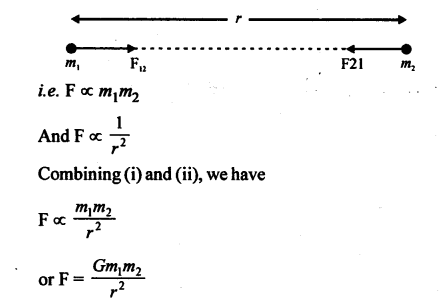Where G is the constant of proportionality and is known as the universal gravitational constant. Its value at all places in this universe is 6.67 × 10-11 Nm2 kg-2.

Question 2.
How is acceleration due to gravity related to
(1) mass of a planet
(2) distance of body from the center of earth?
Acceleration due to gravity : The acceleration of a freely falling body, under the action of gravity of earth, is called acceleration due to gravity.
Consider a body of mass ‘m’ on the surface of earth such that it falls towards it with an acceleration ‘g’.
Let, M = mass of the earth
d = distance between center of earth and center of the body
F = Force acting on the bodyFrom this equation, it is clear that
(1) g ∝ M
Acceleration due to gravity on the earth is directly proportional to the mass of that earth.

(2)Acceleration due to gravity is inversely proportional to square of the distance of the body from centre of the earth.

Question 3.
(a) What do you understand by the term mass?
(b) State two important characteristics of mass.
(c) State units of mass in CGS and SI systems.
(d )Name the device used for measuring mass.
(a) Mass: The quantity of matter contained in a body is known as its mass.
(b) Characteristics of mass :

1. It is independent of the position and surrounding of a body.
2. It is a scalar quantity.
3. It remains constant at all places, provided the velocity of the body is not too high.

(c) CGS unit of mass is gram (g).
SI unit of mass is kilogram (kg).
(d) Physical balance is used to measure the mass of a body.

Question 4.
(a) What do you understand by the term weight?
(b) State two important characteristics of weight
(c) State the units of weight in CGS and SI system.
(d) Name the device used for measuring weight
(a) Weight: Weight of a body is defined as the force with which the earth attracts it.
(b) Characteristics of weight :

1. It depends upon the position and surroundings of body.
2. It is a vector quantity.
3. If changes from place to place on the surface of earth due to the change in the value of acceleration due to gravity (g).

(c) In CGS system, unit of weight is dyne. In SI system, unit of weight is newton (N).
(d) Spring balance is used to measure the weight of the body.

Question 5.
State four differences between mass and weight.
Mass

1. It is the quantity of matter contained in a body.
2. It is constant quantity.
3. It is a scalar quantity.
4. Its unit is kg.
5. Beam balance is used to measure it.
6. At no place its value is zero.

Weight:

1. It is the force with which a body is attracted towards the center of the earth.
2. W = m x g, hence its value changes from place to place according to the value of ‘g’
3. It is a vector quantity.
4. Its unit is ‘newton’.
5. Spring balance is used to measure it.
6. At the center of earth its value is zero.

Question 6.
Does a body weigh same at all places of the Earth? Give a reason for your answer.
No, A body does not weigh same at all places of the earth because value of acceleration due to gravity (g) is different at different places on the surface of earth.

Question 7.
Why is gold not weighed by a spring balance?
Spring balance measures the weight of a body. Weight of a body changes from place to place on the surface of earth due to change in value of acceleration due to gravity. That is why gold is not weighed by a spring balance.

Question 8.
A man sits in a machine which generates acceleration five times more than acceleration due to gravity. If the mass of man is 80 kg, what is his weight? Take g = 10 ms-2.
Mass of the man = m = 80 kg
Acceleration due to gravity = g=10 ms-2
Acceleration generated by the machine
= a = 5 g= 5 x 10 = 50 ms 2
Case-I:
When machine accelerates downward
Effective weight of the man = mg – ma = m(g- a)
= 80 (50-10) = 3200 N
Case-II : When the machine accelerates upward
Effective weight of man = mg + ma = m (g + a)
= 80 (10 + 50)
= 80 x 60 = 4800 N

Question 9.
A man weighs 800 N the at the equator. How does the weight of man change at the following places?
(a) At poles
(b) 100 km up in space
(c) 10 km down in a mine.
Weight of the man at equator = 800 N
(a) At poles : As value of g increases at poles as compared to that at equator, so weight of the body at poles will be more than 800 N.
(b) As the value of g decreases with increase in height, so weight of the man 100 km up in the space is less than 800 N.
(c) As value of g decreases with increase in depth, so weight of the man 10 km down in a mine is less than 800 N.

Question 10.
How is weight affected in the following cases, when initially the body is weighed in Delhi with a spring balance?

1. Body is taken to Moscow.
2. Body is taken to Ceylon.
3. Body is taken to sea level.
4. Body is taken to a high mountain.
5. Body is taken deep inside a mine.

1. When body is taken to Moscow, then weight of the body weighs slightly more than Delhi.
2. When body is taken to Ceylon, then weight of the body is slightly more than at Delhi.
3. When body is taken to sea level, then its weight remains same.
4. As the value of g increases with increase in height of body from surface of the earth, so when a body is taken .to a high mountain, its weight decreases.
5. As the value of g decreases with depth, so when body is taken deep inside a mine, its weight decreases.

Question 11.
Describe, briefly how can you calculate the value of ‘g’ with a simple pendulum.
Simple pendulum : A simple pendulum consists of a small heavy mass in the form a bob suspended by a light inelastic string.
The pendulum is suspended from a suitable support (the thread may be held firmly between two halves of a cork held by a clamp and stand). The pendulum is allowed to oscillate and its time period for one oscillation is noted with the help of a stop­watch by observing a large number of oscillations. The length of the pendulum is changed several times and the time period is determined in each case. A graph is plotted between l, the length of the pendulum, and T2, the square of time period.More Resources

Hope given  A New Approach to ICSE Physics Part 1 Class 9 Solutions Law of Motion are helpful to complete your science homework.

If you have any doubts, please comment below. APlusTopper try to provide online science tutoring for you.